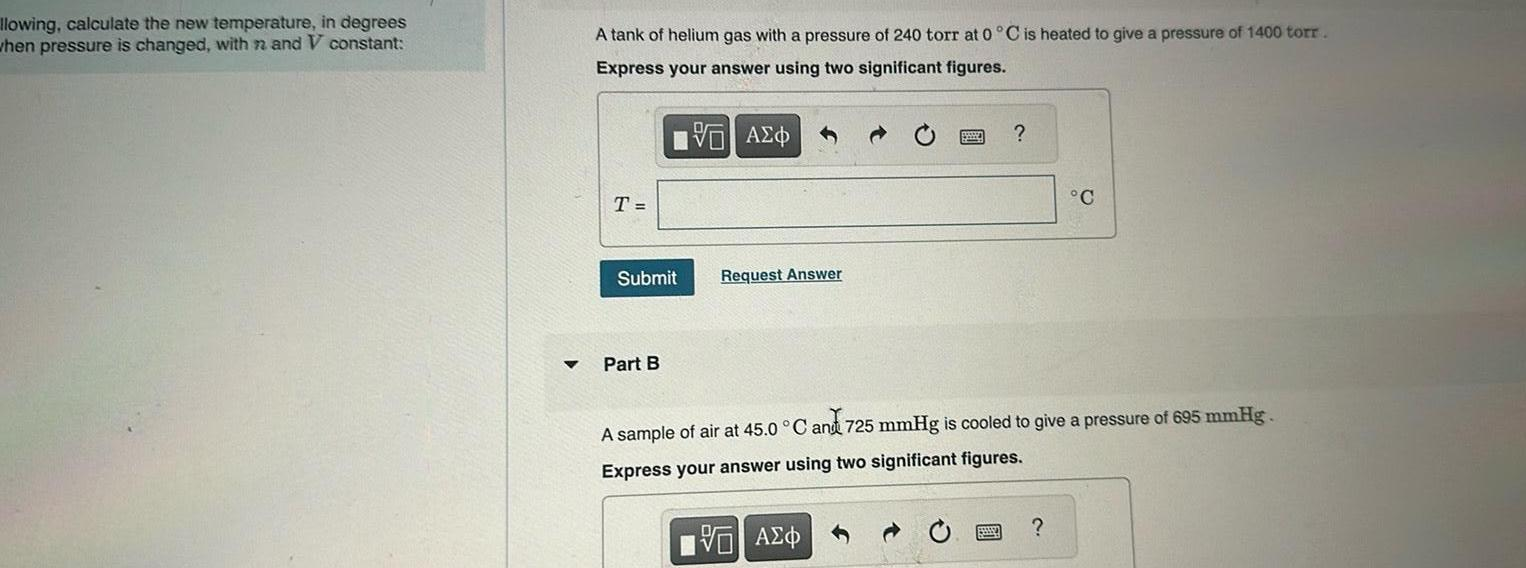Question:

# llowing calculate the new temperature in degrees when

Last updated: 11/19/2023llowing calculate the new temperature in degrees when pressure is changed with n and V constant A tank of helium gas with a pressure of 240 torr at 0 C is heated to give a pressure of 1400 torr Express your answer using two significant figures T Submit Part B AEO Request Answer C A sample of air at 45 0 C and 725 mmHg is cooled to give a pressure of 695 mmHg Express your answer using two significant figures VAE